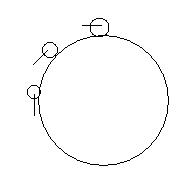# How to use raycast here?

I am using a picture here to explain problem.I want my raycast to look like this when I move around the sphere. Should I use rotation or something else.

Yes, you can use rotation of 90 degrees on the vector that connects your position with the center of the circle. Or you can just use the direction of your velocity if you use physics (in circular motion, the velocity is always 90 degrees to the radius).

This code will work if the circle is on the X/Z plane, and is attached to the object that moves around the circle, where "circleCenter " is a vector representing the position of the center of the circle.

``````Vector3 toCenter = circleCenter - transform.position;
Quaternion rotate90degrees = Quaternion.AngleAxis(90, Vector3.up);
Vector3 raycastDirection = rotate90degrees * toCenter;
Physics.Raycast(transform.position, raycastDirection);
``````

If the small sphere is going to be arbitrarily walking around the big sphere, then you either need to use local coordinates to the small sphere, or you have to have a point of reference. The general solution for sphere walking is to align the small sphere with a vector between center of the large sphere and the center of the small sphere, so that the small sphere is standing ‘up’ at its position on the sphere. Then you can use the transform.forward of the small sphere for your raycast.

``````var q = Quaternion.FromToRotation(smallSphere.up, smallSphere.position-bigSphere.position)
smallSphere.rotation = q * smallSphere.rotation;
if (Physics.Raycast(smallSphere.position, smallSphere.forward)) {
``````

If you have a point of reference, then you can use Vector3.Cross() to find the vector. The point of reference is the axis the small sphere is using when it rotates around the big sphere. The point of reference might be a world vector like Vector3.forward, or it could be related to the position of the camera with respect to the sphere. To calculate the raycast:

``````var rayDir = Vector3.Cross(axis, smallSphere.position - bigSphere.position);
if (Physics.Raycast(smallSphere.position, rayDir)) {
``````

Note to get the raycast in the direction you expect, you might have to either negate the axis or swap the two parameters in the Vector3.Cross().